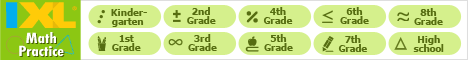Java Games: Flashcards, matching, concentration, and word search.Multiplication Facts - the more difficult facts through 10x10.

This is a review of all the more difficult multiplication facts to 10 x 10. The easier facts are not included.

This activity helps fulfill the following New York State Math Learning Standards (revised 2005) for 3rd grade: 3.N.7, 3.N.8, 3.N.19

AB
2 x 7 =14
2 x 8 =16
2 x 9 =18
2 x 10 =20
3 x 6 =18
3 x 7 =21
3 x 8 =24
3 x 9 =27
3 x 10 =30
4 x 6 =24
4 x 7 =28
4 x 8 =32
4 x 9 =36
4 x 10 =40
5 x 4 =20
5 x 5 =25
5 x 6 =30
5 x 7 =35
5 x 8 =40
5 x 9 =45
5 x 10 =50
6 x 3 =18
6 x 4 =24
6 x 5 =30
6 x 6 =36
6 x 7 =42
6 x 8 =48
6 x 9 =54
6 x 10 =60
7 x 3 =21
7 x 4 =28
7 x 5 =35
7 x 6 =42
7 x 7 =49
7 x 8 =56
7 x 9 =63
7 x 10 =70
8 x 3 =24
8 x 4 =32
8 x 5 =40
8 x 6 =48
8 x 7 =56
8 x 8 =64
8 x 9 =72
8 x 10 =80
9 x 2 =18
9 x 3 =27
9 x 4 =36
9 x 5 =45
9 x 6 =54
9 x 7 =63
9 x 8 =72
9 x 9 =81
9 x 10 =90
10 x 6 =60
10 x 7 =70
10 x 8 =80
10 x 9 =90
10 x 10 =100
0 x 7 =0
0 x 8 =0
0 x 9 =0
0 x 10 =0
6 x 0 =0
7 x 0 =0
8 x 0 =0
9 x 0 =0
10 x 0 =0This activity was created by a Quia Web subscriber. Learn more about QuiaCreate your own activities